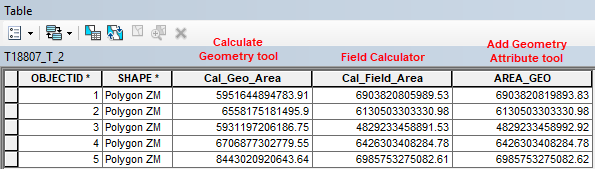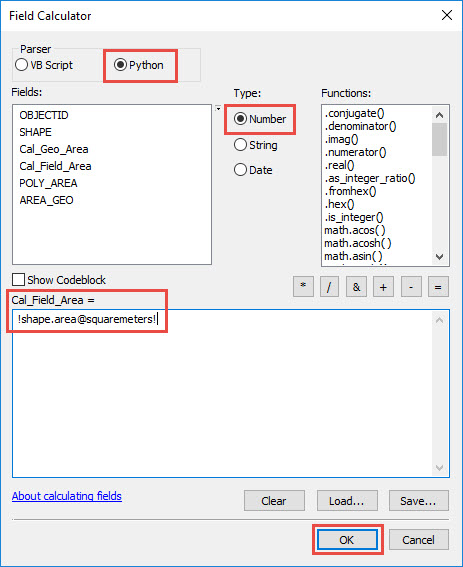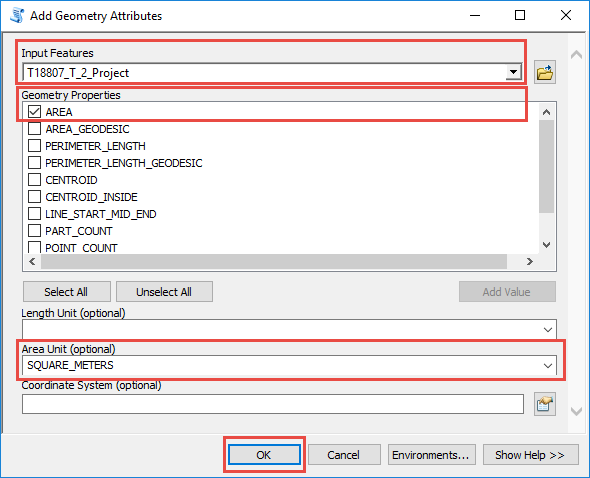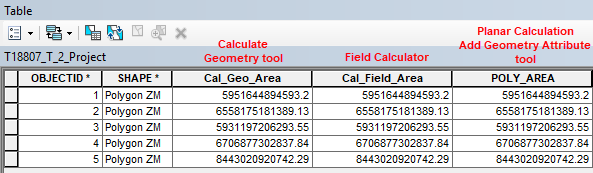English

# Problem: The Calculate Geometry tool returns different area results than the Field Calculator and Add Geometry Attribute tools

## Description

In ArcMap, when using the Calculate Geometry tool to calculate areas of a feature layer, the output in the attribute table is different compared to the areas calculated by the Field Calculator and the Add Geometry Attribute tools. The same issue also occurs when calculating the length of line features and perimeters of polygon features.## Cause

• The dataset uses a geographic coordinate system (GSC) while the data frame uses a projected coordinate system (PCS). The Calculate Geometry tool defaults to the coordinate system used by the data frame. The Calculate Geometry tool only returns the correct results when the dataset is projected. This is because the Calculate Geometry tool uses planar geometry for calculations. The Field Calculator and Add Geometry Attribute tools use geodesic geometry for calculation. For more information, refer to ArcMap Web Help: Fundamentals of field calculations.
• When both the dataset and the data frame use a PCS but of different coordinate systems, the output may vary slightly. This is due to the variation in the spatial properties and distortions of the different coordinate systems. For more information, refer to ArcMap Web Help: Calculating area, length, and other geometric properties.

## Solution or Workaround

Use the Field Calculator and the Add Geometry Attribute tool to calculate areas and lengths of feature layers after projecting the dataset to a PCS.

Use a Python script in the Field Calculator with the dataset in a PCS

1. In the attribute table, right-click the header of the desired field and click Field Calculator.
2. Select Python parser and Number type.
3. To calculate the area, type the following expression for the field and click OK. For more information on the areal and linear measure keywords, refer to Geometry unit conversions.
`!shape.area@unit! , for example !shape.area@squaremeters!`Use the Add Geometry Attribute tool
1. In the Catalog window, navigate to Data Management Tools > Features > Add Geometry Attributes.
2. In the Add Geometry Attribute dialog box, set Input Features.
3. For Geometry Properties, check AREA and set desired Area Unit. Click OK and view the results in the attribute table.
```Note:
The AREA property uses planar calculation whereas AREA_GEODESIC uses geodesic calculation. For more information refer to ArcMap Web Help: Add Geometry Attributes.``````Note:
The best practice to obtain accurate results is to project the dataset from the GCS to a PCS used by the data frame. For more information refer to ArcMap Web Help: Project.```